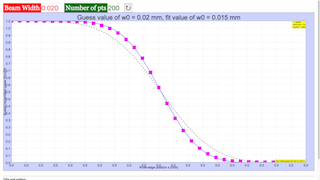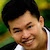Intro Page

Laser Beam Profile

Developed by E. Behringer

This set of exercises guides the student to model the results of an experiment to determine the profile of a laser beam using a knife-edge technique. It requires the development of the model of the knife-edge profile, and fitting of the model profile to experimental data. Here, the computational tasks are handled by built-in functions of the computational tool being used to complete these exercises.

 Subject Area Waves & Optics Beyond the First Year Python Students who complete this set of exercises will be able to express an equation predicting the profile of a laser oscillating in the TEM00 mode in terms of dimensionless (“scaled”) variables suitable for coding (Exercise 1); produce both line plots and contour plots of the (scaled) irradiance of the beam versus (scaled) position(s) (Exercises 1 and 2); develop a model of, and plot, the knife-edge profile of the laser beam (Exercise 3); and fit the model, i.e., of the irradiance versus knife-edge position, to experimental data (Exercise 4) 120 min
Exericse 4

### EXERCISE 4: FIT EXPERIMENTAL DATA TO THE MODEL KNIFE-EDGE PROFILE

Imagine that you have gone to the lab, set up the experiment to determine the beam profile, and obtained the data shown in the table below. Uncertainties in $x$ are $\mathrm{\Delta }x=±0.0001$ in., and the uncertainties in $P\left(x\right)/{P}_{0}$are $±0.001$.

$x$ [in.] $P\left(x\right)/{P}_{0}$ $x$ [in.] $P\left(x\right)/{P}_{0}$
-0.0366 1.000 0.0014 0.420
-0.0346 1.000 0.0034 0.315
-0.0326 1.000 0.0054 0.229
-0.0306 0.999 0.0074 0.156
-0.0286 0.999 0.0094 0.105
-0.0266 0.998 0.0114 0.066
-0.0246 0.998 0.0134 0.040
-0.0226 0.998 0.0154 0.025
-0.0206 0.995 0.0174 0.016
-0.0186 0.992 0.0194 0.012
-0.0166 0.983 0.0214 0.010
-0.0146 0.968 0.0234 0.008
-0.0126 0.947 0.0254 0.007
-0.0106 0.915 0.0274 0.007
-0.0086 0.870 0.0294 0.007
-0.0066 0.814 0.0314 0.006
-0.0046 0.738 0.0334 0.006
-0.0026 0.638 0.0354 0.005
-0.0006 0.532

On the same graph, plot: the experimental data with error bars: the “guess” function  with mm; and the fit function.

Beam_Profile_Exercise_4.py

#

# Beam_Profile_Exercise_4.py

#

# This file will generate a plot of

# beam profile data, guess function, and fit function

# for the TEM00 laser mode

#

# The fit is generated using the curve_fit function of Python

#

# Written by:

#

# Ernest R. Behringer

# Department of Physics and Astronomy

# Eastern Michigan University

# Ypsilanti, MI 48197

# (734) 487-8799

# This email address is being protected from spambots. You need JavaScript enabled to view it.

#

# Selected and normalized power data from graduate student:

#

# Najwa Sulaiman

# Department of Physics and Astronomy

# Eastern Michigan University

# Ypsilanti, MI 48197

# This email address is being protected from spambots. You need JavaScript enabled to view it.

#

# 20160627 by ERB

#

# import the commands needed to make the plot and fit the data

from __future__ import print_function

from pylab import xlim,xlabel,ylim,ylabel,grid,show,plot,legend,title

from numpy import linspace,ones,sqrt

from scipy.special import erf

from scipy.optimize import curve_fit

from matplotlib.pyplot import errorbar

# Inputs

npts_guess = 200 # number of intervals in the guess function

#

# Initialize the fit parameter(s)

w0 = 0.020 # The initial guess for w0 [in.]

print('The initial guess for w0 is ',w0,' in.')

# The knife-edge position data (corrected from raw data) [in.]

Xcorr_points = -linspace(0.0366, -0.0354, 37)

# The power data (corrected and normalized from raw data)

Powercorr_points = [1.000, 1.000, 1.000, 0.999, 0.999, 0.998, 0.998, 0.998, 0.995, 0.992, 0.983, 0.968, 0.947, 0.915, 0.870, 0.814, 0.738, 0.638, 0.532, 0.420, 0.315, 0.229, 0.156, 0.105, 0.066, 0.040, 0.025, 0.016, 0.012, 0.010, 0.008, 0.007, 0.007, 0.007, 0.006, 0.006, 0.005]

# Error bar data here

Xcorr_points_err = 0.0001*ones(len(Xcorr_points)) # position error [in.]

Powercorr_points_err = 0.01*ones(len(Powercorr_points)) # scaled power error

# Define the fit function: 0.5*(1.0 - erf(sqrt(2.0)*x/w0))

def func(x, w0):

return 0.5*(1.0 - erf(sqrt(2.0)*x/w0))

# Generate the guess function

# First, the knife-edge position values

Xcorr_inch_guess = linspace(min(Xcorr_points),max(Xcorr_points),npts_guess)

# Second, the scaled power guess

Powercorr_guess = func(Xcorr_inch_guess, w0)

# Use the curve_fit function to fit the model to the experimental data

# Note that the output consists of two parts:

# The first part is an array of the values of the fit parameters

# as determined by a least-squares fitting algorithm;

# the second part is the covariance array (a square, symmetric matrix)

# that has the uncertainties in the parameters on the diagonal of the array.

curve_fit_output = curve_fit(func, Xcorr_points, Powercorr_points, w0)

print(curve_fit_output)

w0_fit = curve_fit_output

# Generate the array needed to make a smooth plot of the fit function

Powercorr_fit = func(Xcorr_inch_guess,w0_fit)

# Define the limits of the horizontal axis

xlim(min(Xcorr_points),max(Xcorr_points))

# Label the horizontal axis, with units

xlabel("Knife-edge position $$x$$ [inch]", size = 16)

# Define the limits of the vertical axis

ylim(min(Powercorr_points),max(Powercorr_points))

# Label the vertical axis, with units

ylabel("Scaled transmitted power $$P(x)/P_0$$", size = 16)

# Make a grid on the plot

grid(True)

# Plot the data as symbols with error bars: magenta (m) circles (o).

errorbar(Xcorr_points,Powercorr_points,xerr=Xcorr_points_err,yerr=Powercorr_points_err,fmt="mo",label="Data")

# Plot the guess as a line: black (k) dashed (--)

plot(Xcorr_inch_guess,Powercorr_guess,"k--",label="Guess")

# Plot the fit as a line: blue (b)

plot(Xcorr_inch_guess,Powercorr_fit,"b",label="Fit")

# Make the legend

legend(loc=1)

# Make title

title('Guess value of $$w_0 =$$%.2e mm, fit value of $$w_0 =$$%.2e mm'%(w0,w0_fit))

show()

### Translations

Code Language Translator Run### Software Requirements

SoftwareRequirements

 Android iOS Windows MacOS with best with Chrome Chrome Chrome Chrome support full-screen? Yes. Chrome/Opera No. Firefox/ Samsung Internet Not yet Yes Yes cannot work on some mobile browser that don't understand JavaScript such as..... cannot work on Internet Explorer 9 and below

### CreditsFremont Teng; Loo Kang Wee

[text]

[text]

Research

[text]

[text]

[text]